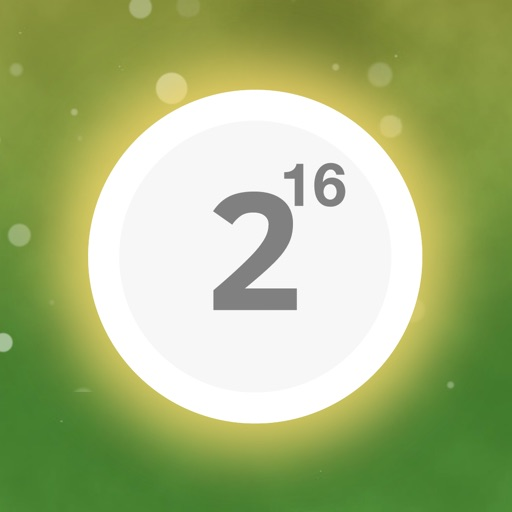## Join the numbers and get to the 65536 to win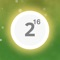# 65536 - Ultimate Challenge Puzzle Game Free

by Xuehui Wu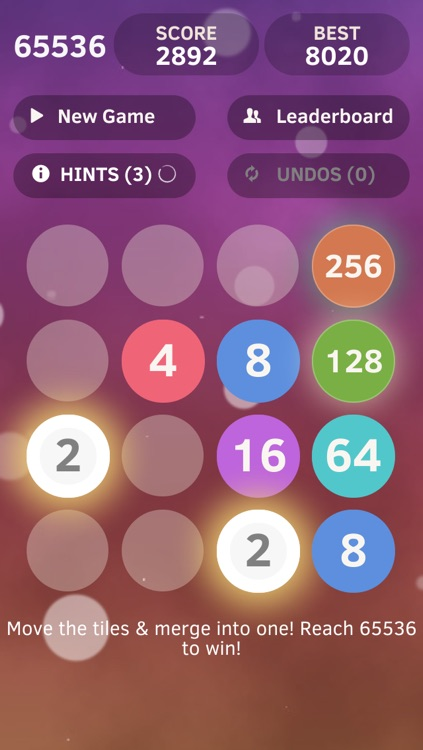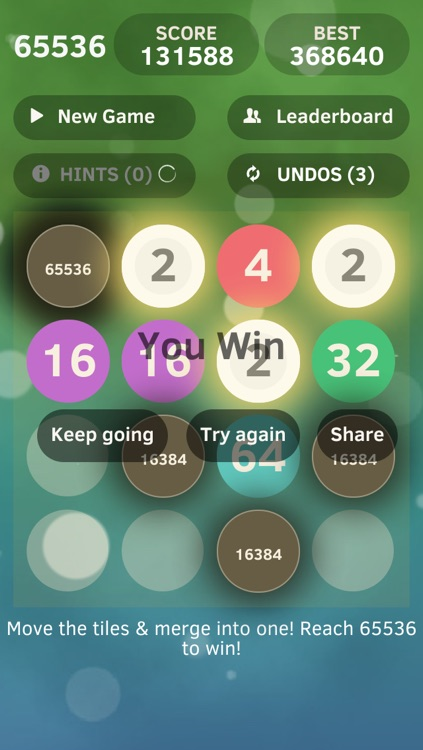Join the numbers and get to the 65536 to win! It is the Highest Possible Number for 2048 puzzle game ever! Is it impossible to reach? The 3 hints & 3 undos will help you out. Get it & start your challenge now!!!! =)Version
1.3
Rating
NA
Size
5Mb
Genre
Action Puzzle
Last updated
August 11, 2014
Release date
April 29, 2014

### App Screenshots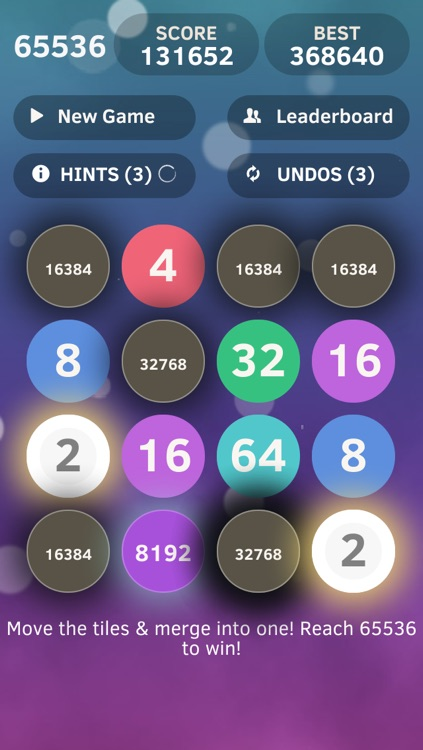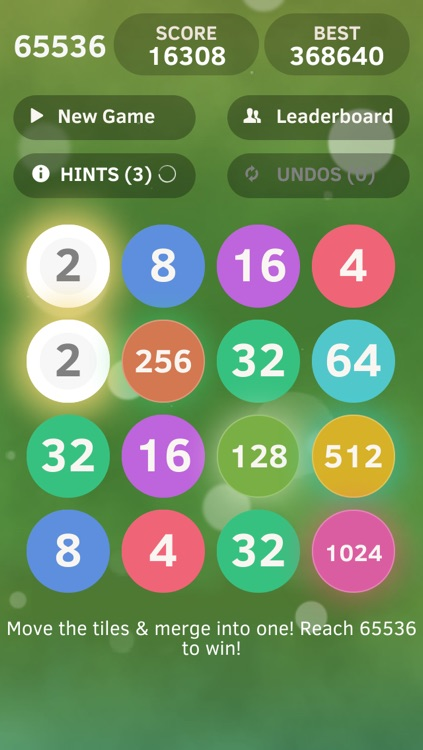### App Store Description

Join the numbers and get to the 65536 to win! It is the Highest Possible Number for 2048 puzzle game ever! Is it impossible to reach? The 3 hints & 3 undos will help you out. Get it & start your challenge now!!!! =)

---------------------------
Highest possible score for 2048
---------------------------

Instead of playing the game (and being somewhat consumed by it), I figured I might as well work out the highest possible score.
This assumes that every tile you spawn is a 2, and the last tile you spawn is a 4.
There are 16 squares. You'd think that the highest possible number would be 216 (65536), which would fill up the board, making it impossible to progress. However, if you do manage to spawn a 4 tile as your last tile, then you can continue to progress to 217 (131972).
Scoring works like this;
Merging two lower tier blocks together will give you the score of the higher tier block (score of +8 gained from merging two 4's). For any specific tile score, you have to add up all the scores from the lower tiers.
Creating a 2 tile = +0pts
Creating a 4 tile = 4 = +4pts
8 = 8 + 2x4 = +16pts
16 = 16 + 2x8 + 4x4 = +48pts
32 = 32 + 2x16 + 4x8 + 8x4 = +128pts ...
Which can be simplified;
Tile 21 = 0 x 21 = 0pts
Tile 22 = 1 x 22 = 4pts
Tile 23 = 2 x 23 = 16pts
Tile 24 = 3 x 24 = 48pts
Making tile 2n = (n-1)2n points.
Making the max tile (217 ) = 16 x 217 = 2,097,152 points. You would need to spawn 65,536 '2' tiles to make a single 131,072 tile, which, at 1 legal move/s, would take 18.2 hours.
That's only making the max tile now. we still need to fill the rest of the board. (Note: combining times are correct, as when you merge 2 tiles together, you spawn a '2' at the same time)
Next tile 216 = 983,040 points (215 tiles/9.1 hours)
215 = 458,752 points (214 tiles/4.55 hours)
214 = 212,992 points (213 tiles/2.27 hours)
213 = 98,304 points (212 tiles/1.13 hours)
212 = 45,056 points (211 tiles/34 mins)
211 (2048) = 20,480 points (210 tiles/17 mins)
1024 = 9,216 points (29 tiles/8.5 mins)
512 = 4,096 points (28 tiles/4.3 mins)
256 = 1,792 points (27 tiles/2.1 mins)
126 = 768 points (26 tiles / 64 seconds)
64 = 320 points (25 tiles /32 seconds)
32 = 128 points (16 tiles/16 seconds)
16 = 48 points (8 tiles/8 seconds)
8 = 12 points (3 tiles/3 seconds) [This space will be created by two '2' tiles, and the last '4' tile. so -4 points from the equation]
This fills up 15/16 spaces on the board. We don't include the last tile, as it cannot merge with the 8.
Max points possible = 3,932,156 Points. 131,070 (+1 for last tile, -1 for the 4) tiles spawned. Around 35.5 hours @ 1 move/s.
If you happen to miss out on the last '4' tile, your max score would be 1,835,008, 65,535 (+1 for last tile) '2' tiles spawned, 18.2 hours @ 1 move/s.